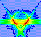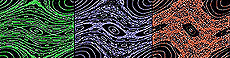Standard Map Main Page Previous | ContinueWhat is a Map? The word "map" in the phrase "Standard Map" has a different meaning than it does in everyday life. It doesn't mean that the Standard Map is a chart or a guide to a location. In physics and mathematics, a "map" is any mathematical transformation that is applied over and over again in sequence. Sometimes the "mapping" is used instead of "map."Standard Map Main Page Previous | ContinueWhat is a Map? The word "map" is used as a verb too. A physicist might say that under a particular mathematical transformation, a certain point is "mapped" to another point at a different location. The "map" equations can be used over and over again on a single point, so that the point gets "mapped" repeatedly to new locations. Each implementation of the mathematical equations is called an iteration of the map.Standard Map Main Page Previous | ContinueWhat is a Map? The best way to see this is by an example. First let's make up a starting condition, then we'll make up a "map." Suppose that you are keeping track of the location of a point by the variable x. At the beginning, suppose that the location is 2. In other words, initially, we have: ``` x = 2 ``` The value of the starting point isn't important here. We just picked "2" to give an example.Standard Map Main Page Previous | ContinueWhat is a Map? Now that we have our starting condition, let's make up a map. An example of a very simple map might be expressed as: "To get the location of the new point, add three to location of the old point." The way you would write this map in mathematical terms is: ``` x' = x + 3 ``` The map above is not a very exciting map, to be sure, but is useful for showing how all maps work, including the Standard Map. Notice the way that this map is written in mathematical form. There are two variables present, x and x', which is pronounced "x prime." In this case, x represents the old location of the point, and x' represents the new location of the point, after the map is iterated. Using primed variables like this is a very common way of writing the mathematical equations of maps, by the way.Standard Map Main Page Previous | ContinueWhat is a Map? So now we have a starting condition, and the map to go with it. Let's see what happens when we apply the mapping equation to the starting condition. We started out with: ``` x = 2 (Starting condition) x' = x + 3 (The map) ``` When we iterate the map, the effect can be shown by a column of numbers as follows: ``` x ---> x' 2 ---> 5 (First iteration) 5 ---> 8 (Second iteration) 8 ---> 11 (Third iteration) 11 ---> 14 (Fourth iteration) . (etc.) . . ``` Notice that as promised, the effect of this particular map is to add 3 to value of the old point to get the new point. Notice another important point: after the map is iterated, the "new" value (x-prime) becomes the "old" value for the next time that the map is iterated. The dots at the bottom of the column mean that you could keep on iterating the map forever, adding three to the old value over and over again.Standard Map Main Page Previous | ContinueWhat is a Map? So that's basically how maps work in general. In the next section, you will learn how multi-dimensional maps work.